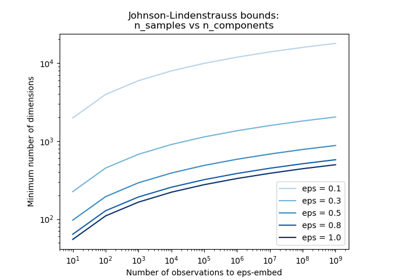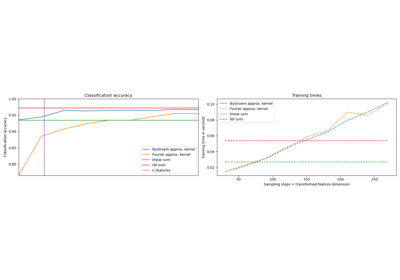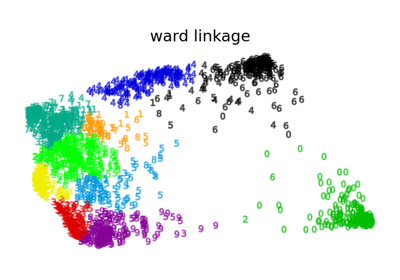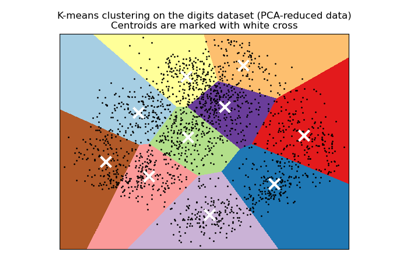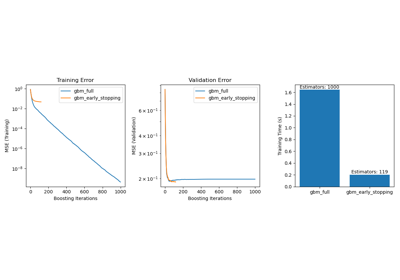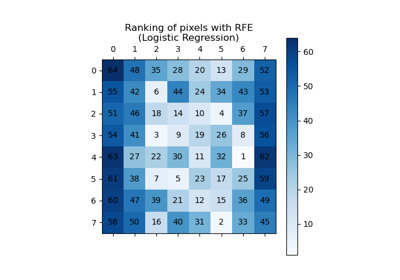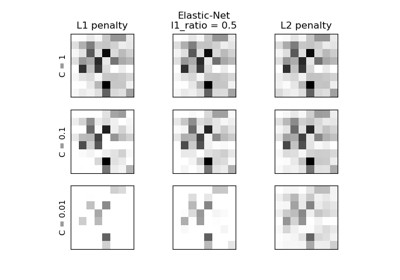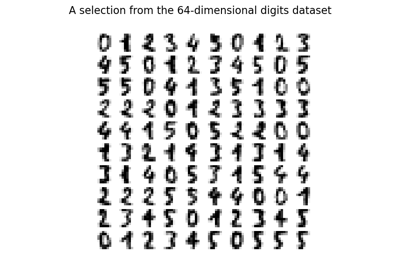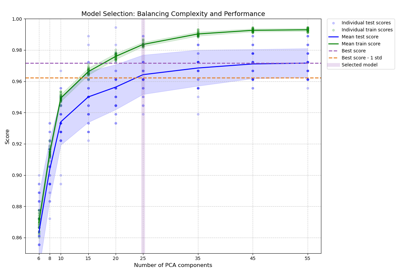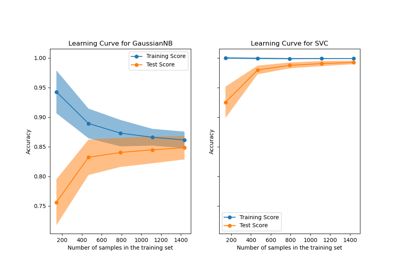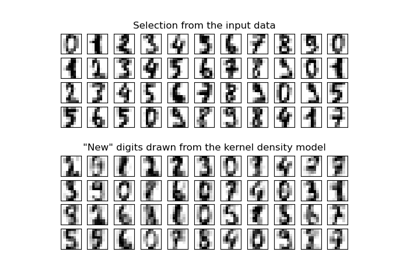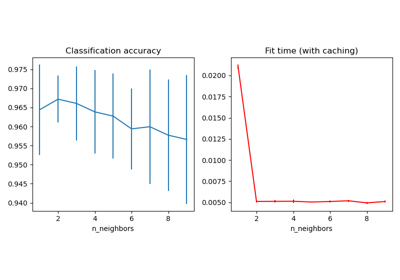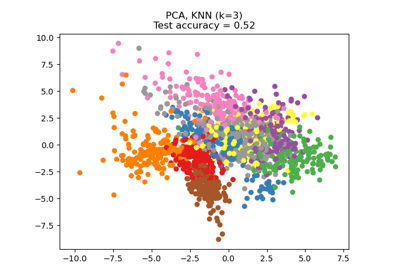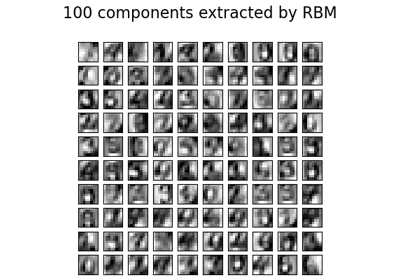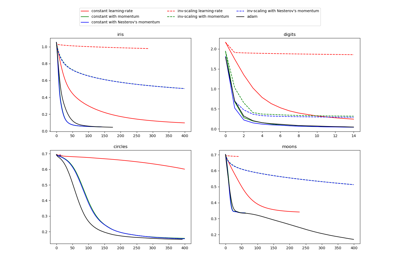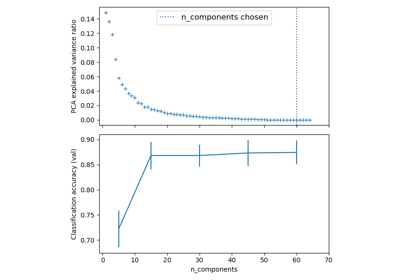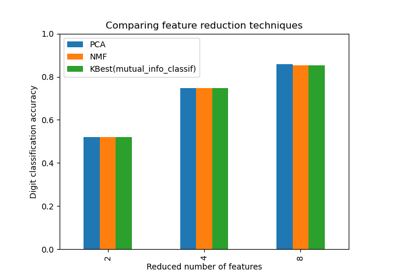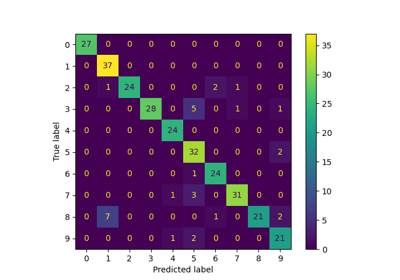# sklearn.datasets.load_digits¶

sklearn.datasets.load_digits(n_class=10, return_X_y=False)[source]

Load and return the digits dataset (classification).

Each datapoint is a 8x8 image of a digit.

 Classes 10 Samples per class ~180 Samples total 1797 Dimensionality 64 Features integers 0-16

Read more in the User Guide.

Parameters
n_classinteger, between 0 and 10, optional (default=10)

The number of classes to return.

return_X_yboolean, default=False.

If True, returns (data, target) instead of a Bunch object. See below for more information about the data and target object.

New in version 0.18.

Returns
dataBunch

Dictionary-like object, the interesting attributes are: ‘data’, the data to learn, ‘images’, the images corresponding to each sample, ‘target’, the classification labels for each sample, ‘target_names’, the meaning of the labels, and ‘DESCR’, the full description of the dataset.

(data, target)tuple if return_X_y is True

New in version 0.18.

This is a copy of the test set of the UCI ML hand-written digits datasets
https://archive.ics.uci.edu/ml/datasets/Optical+Recognition+of+Handwritten+Digits

Examples

To load the data and visualize the images:

>>> from sklearn.datasets import load_digits
>>> print(digits.data.shape)
(1797, 64)
>>> import matplotlib.pyplot as plt
>>> plt.gray()
>>> plt.matshow(digits.images)
>>> plt.show()


## Examples using sklearn.datasets.load_digits¶